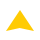The math curriculum
MINI CRITIQUE - Isagani Cruz (The Philippine Star) - August 12, 2015 - 10:00am

Last week in Singapore, Filipino students from public and private elementary and secondary schools in the Philippines beat China and Taiwan in the 2015 Singapore International Math Competition.

Earlier, the Philippines placed second in the China Mathematics Olympiad, losing only to China, but beating Hong Kong, Thailand, Taiwan, and Macau.

The victories can be credited partly to the Mathematics Trainers Guild Philippines (MTG), which conducted national competitions to choose the members of the Philippine team.

Much of the credit, however, goes to the Department of Education (DepEd), which radically changed the mathematics curriculum to conform to the goals of the K to 12 reform.

We should remember that, in the international Trends in Mathematics and Science Study, the Philippines was always close to the bottom of the rankings. In 1999 and 2003, we were third from the bottom.

How has the turn-around been achieved?

Let us look closely at the mathematics curriculum of K to 12. Remember that, since June 2012, elementary school and secondary school learners have been using the new K to 12 curriculum. (DepEd prefers the term “learners” to “pupils” or “students.”)

The big change is the spiraling of content. Today, learners learn all the areas of mathematics starting in Kindergarten. The learning is enhanced little by little as the learners go up the ladder of basic education.

Here are the standards for Kindergarten to Grade 3: “At the end of Grade 3, the learner demonstrates understanding and appreciation of key concepts and skills involving numbers and number sense (whole numbers up to 10,000 and the four fundamental operations, including money, ordinal numbers up to 100th, basic concepts of fractions); measurement (time, length, mass, capacity, area of square and rectangle); geometry (2-dimensional and 3-dimensional objects, lines, symmetry, and tessellation); patterns and algebra (continuous and repeating patterns and number sentences); statistics and probability (data collection and representation in tables, pictographs and bar graphs and outcomes) as applied – using appropriate technology – in critical thinking, problem solving, reasoning, communicating, making connections, representations, and decisions in real life.”

Note that algebra, geometry, and statistics (previously high school subjects) are taught as early as Kindergarten.

The standards from Grade 4 to Grade 6 are: “At the end of Grade 6, the learner demonstrates understanding and appreciation of key concepts and skills involving numbers and number sense (whole numbers, number theory, fractions, decimals, ratio and proportion, percent, and integers); measurement (time, speed, perimeter, circumference and area of plane figures, volume and surface area of solid/space figures, temperature and meter reading); geometry (parallel and perpendicular lines, angles, triangles, quadrilaterals, polygons, circles, and solid figures); patterns and algebra (continuous and repeating patterns, number sentences, sequences, and simple equations); statistics and probability (bar graphs, line graphs and pie graphs, simple experiment, and experimental probability) as applied – using appropriate technology – in critical thinking, problem solving, reasoning, communicating, making connections, representations, and decisions in real life.”

Note the repetition of the topics. Spiraling means that the same topics are taught in every grade level, but with increasing complexity.

The standards for Grade 7 to Grade 10 are: “At the end of grade 10, the learner demonstrates understanding and appreciation of key concepts and skills involving numbers and number sense (sets and real numbers); measurement (conversion of units); patterns and algebra (linear equations and inequalities in one and two variables, linear functions, systems of linear equations, and inequalities in two variables, exponents and radicals, quadratic equations, inequalities, functions, polynomials, and polynomial equations and functions); geometry (polygons, axiomatic structure of geometry, triangle congruence, inequality and similarity, and basic trigonometry); statistics and probability (measures of central tendency, variability and position; combinatorics and probability) as applied – using appropriate technology – in critical thinking, problem solving, communicating, reasoning, making connections, representations, and decisions in real life.”

In Grade 11, the required core subject named “General Mathematics” focuses a bit more on the “real life” phrase that occurs at the end of the standards above. By the end of Grade 11, the learner, for example, should be able to “accurately construct mathematical models to represent real-life situations using functions; investigate, analyze, and solve problems involving simple and compound interests and simple and general annuities using appropriate business and financial instruments; and decide wisely on the appropriateness of business or consumer loans and their proper utilization.”

If the learner opts for the Science, Technology, Engineering, and Mathematics (STEM) Strand of the Academic Track, s/he rounds off the mathematics of earlier grades with “population models and areas of plane regions” in the Basic Calculus subject.

Even if the learner goes into the other Tracks, s/he will be able to meet the College Readiness Standards (CRS) of the Commission on Higher Education (CHED). These CRS include the ability to handle “graphs of linear equations in two variables, polynomial functions, quadrilaterals, permutation, bearing, second derivatives, growth and decay, testing claims about variances, continuous compound interest, and antiderivatives.”

Clearly, the mathematical knowledge and skills in the K to 12 curriculum are much more advanced than in previous curriculums. No wonder our K to 12 learners are making waves in international mathematics competitions abroad.

Philstar
• Latest
Latest